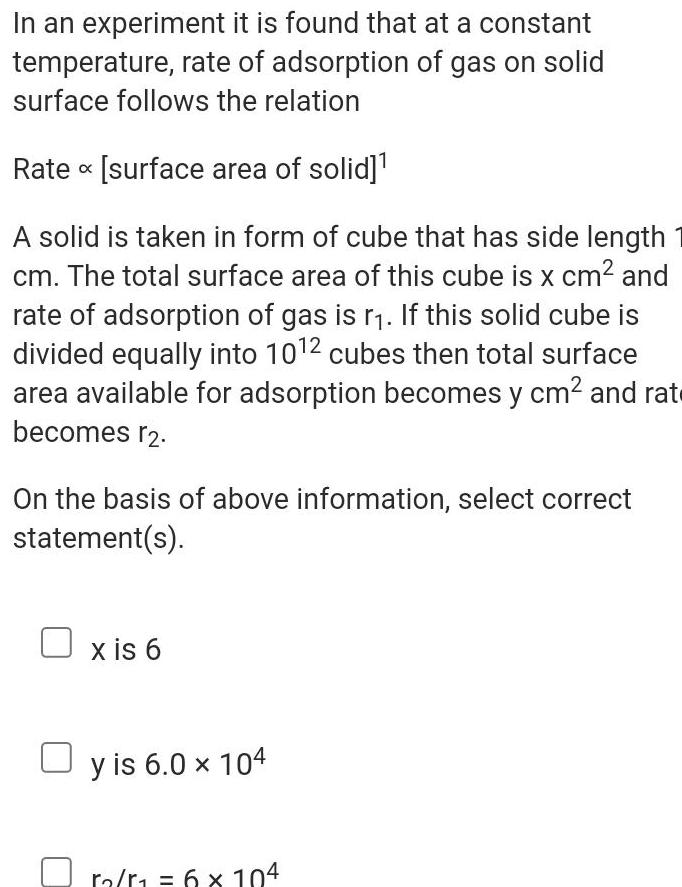Question:

# In an experiment it is found that at a constant temperature

Last updated: 7/4/2023In an experiment it is found that at a constant temperature rate of adsorption of gas on solid surface follows the relation Rate surface area of solid A solid is taken in form of cube that has side length cm The total surface area of this cube is x cm and rate of adsorption of gas is r If this solid cube is divided equally into 10 2 cubes then total surface area available for adsorption becomes y cm and rat becomes r2 On the basis of above information select correct statement s x is 6 y is 6 0 x 104 6 x 104